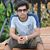BCA 2nd sem Database Management Systems paper 2017

UniversityGGSIPU, New Delhi
CourseBCA
Semester2
SubjectDatabase Management Systems
Year2017
Uploaded ByQuestion Marks 2 requests (a) Write a short note on three scheme architecture.  This question has 0 answers so far. 4.5 0 requests b) Explain different types of Data Independence. This question has 0 answers so far. 3 0 requests (a) Explain the term Generalization and Specialization with suitable example This question has 0 answers so far. 4 0 requests b) Suppose you have a table for a dance studio. The attributes are dancer's identification number, dancer's name, dancer's address, dancer's telephone number, class identification number, day that the class meets, time that the class meets, instructor name, and instructor identification number. Assume that each dancer takes one class, each meets only once a week and has one instructor and each instructor can teach more than one class. Dancer Dancer ID, Dancer_Name, Dancer Address, Dancer Phone, Class ID, Class_Day, Class_Time, Instructor_Name, Instructor ID) Draw an entity- relationship diagram (ERD) for this database. This question has 0 answers so far. 8.5 0 requests (a) Explain the SQL operators BETWEEN, AND, IN, LIKE and IS_NULL by taking suitable examples.  This question has 0 answers so far. 5 0 requests (b) Discus various data types available in SQL. This question has 0 answers so far. 4.5 0 requests (c) SQL allows attributes to have a special value NULL, which is called the null value. What are three common interpretations that can be put on null values?  This question has 0 answers so far. 3 0 requests (a) What is a weak entity sct? What are two principles sources of weak entity sets? Give examples to explain.  This question has 0 answers so far. 6 0 requests (b) What do you understand by referential integrity constraint and attribute-based check constraint? This question has 0 answers so far. 6.5 0 requests (b) Differentiate between Data Definition Language (DDL) and Data Manipulation Language (DML). This question has 0 answers so far. 3 0 requests (c) Write a short note on 3NF.  This question has 0 answers so far. 3 0 requests (a) List the difference between Equijoin and Natural join. Give example of each join operation. This question has 0 answers so far. 6 0 requests (b) What are the problems caused by data redundancies? Can data redundancies be completely eliminated when a database approach is used? Explain this with the help of an example.  This question has 0 answers so far. 6.5 0 requests (a) Describe Two Phase Locking protocol with suitable example.  This question has 0 answers so far. 6 0 requests (b) Describe Deadlock with suitable example and also explain about recovery from the deadlock.  This question has 0 answers so far. 6.5 0 requests Write a short note on (i) fit PrimaryThis question has 0 answers so far. 1 0 requests Write a short note on (ii) Candidate key This question has 0 answers so far. 1 0 requests Write a short note on (iii) Super key This question has 0 answers so far. 1 0 requests Write a short note on (iv) Derived attribute This question has 0 answers so far. 1 0 requests Write a short note on (v) Multivalued attribute This question has 0 answers so far. 1 0 requests Answer the following: (a) What do you mean by functional dependency? Discuss with suitable example.  This question has 0 answers so far. 5 0 requests Answer the following: (b) What is lock? What are the various types of locks used for concurrency control? This question has 0 answers so far. 5 0 requests Answer the following: (c) Describe any four main functions of a database administrator. This question has 0 answers so far. 5 0 requests Answer the following: (d) Define the following terms giving examples for each of them: Entity,attribute, role and relationship between the entities.  This question has 0 answers so far. 5 0 requests Answer the following: (e) Differentiate between database management system and file system. This question has 0 answers so far. 5 0 requests (a) Given the following relations: Vehicle (reg.no, make, colour)Person (eno, name, address) Owner (eno, reg.no) Write expressions in relational algebra to answer the following queries: (i) List the names of persons who do not own any car. This question has 0 answers so far. 3 0 requests (a) Given the following relations: Vehicle (reg.no, make, colour)Person (eno, name, address) Owner (eno, reg.no) Write expressions in relational algebra to answer the following queries: (ii) List the names of persons who own only Maruti Cars,  This question has 0 answers so far. 3 0 requests (a) Given the following relations Vehicle (reg.no, make, colour) Person (eno, name, address) Owner (eno, reg.no) Write expressions in relational algebra to answer the following queries: (ii) list the names of persons who own only Maruti Cars. This question has 0 answers so far. 3 0 requests Write short note on:(a) Time Stamp Based Concurrency Control. This question has 0 answers so far. 4 0 requests Write short note on:(b) Backup and Recovery Techniques  This question has 0 answers so far. 4 0 requests Write short note on:(c) Serializable and Non Scrializable Transactions This question has 0 answers so far. 4.5